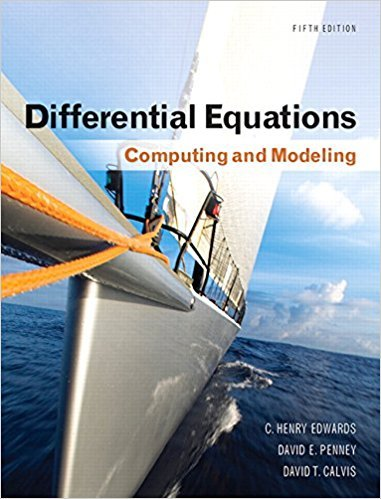×
×

# Solutions for Chapter 5: Linear Systems of Differential Equations## Full solutions for Differential Equations: Computing and Modeling | 5th Edition

ISBN: 9780321816252Solutions for Chapter 5: Linear Systems of Differential Equations

Solutions for Chapter 5
4 5 0 243 Reviews
26
0
##### ISBN: 9780321816252

Since 50 problems in chapter 5: Linear Systems of Differential Equations have been answered, more than 1909 students have viewed full step-by-step solutions from this chapter. Differential Equations: Computing and Modeling was written by and is associated to the ISBN: 9780321816252. This expansive textbook survival guide covers the following chapters and their solutions. This textbook survival guide was created for the textbook: Differential Equations: Computing and Modeling, edition: 5. Chapter 5: Linear Systems of Differential Equations includes 50 full step-by-step solutions.

Key Math Terms and definitions covered in this textbook
• Adjacency matrix of a graph.

Square matrix with aij = 1 when there is an edge from node i to node j; otherwise aij = O. A = AT when edges go both ways (undirected). Adjacency matrix of a graph. Square matrix with aij = 1 when there is an edge from node i to node j; otherwise aij = O. A = AT when edges go both ways (undirected).

• Cofactor Cij.

Remove row i and column j; multiply the determinant by (-I)i + j •

• Commuting matrices AB = BA.

If diagonalizable, they share n eigenvectors.

• Cramer's Rule for Ax = b.

B j has b replacing column j of A; x j = det B j I det A

• Diagonal matrix D.

dij = 0 if i #- j. Block-diagonal: zero outside square blocks Du.

• Diagonalization

A = S-1 AS. A = eigenvalue matrix and S = eigenvector matrix of A. A must have n independent eigenvectors to make S invertible. All Ak = SA k S-I.

• Distributive Law

A(B + C) = AB + AC. Add then multiply, or mUltiply then add.

• Exponential eAt = I + At + (At)2 12! + ...

has derivative AeAt; eAt u(O) solves u' = Au.

• Four Fundamental Subspaces C (A), N (A), C (AT), N (AT).

Use AT for complex A.

• Gram-Schmidt orthogonalization A = QR.

Independent columns in A, orthonormal columns in Q. Each column q j of Q is a combination of the first j columns of A (and conversely, so R is upper triangular). Convention: diag(R) > o.

• Linearly dependent VI, ... , Vn.

A combination other than all Ci = 0 gives L Ci Vi = O.

• Multiplicities AM and G M.

The algebraic multiplicity A M of A is the number of times A appears as a root of det(A - AI) = O. The geometric multiplicity GM is the number of independent eigenvectors for A (= dimension of the eigenspace).

• Nilpotent matrix N.

Some power of N is the zero matrix, N k = o. The only eigenvalue is A = 0 (repeated n times). Examples: triangular matrices with zero diagonal.

• Projection p = a(aTblaTa) onto the line through a.

P = aaT laTa has rank l.

• Reflection matrix (Householder) Q = I -2uuT.

Unit vector u is reflected to Qu = -u. All x intheplanemirroruTx = o have Qx = x. Notice QT = Q-1 = Q.

• Row picture of Ax = b.

Each equation gives a plane in Rn; the planes intersect at x.

• Singular Value Decomposition

(SVD) A = U:E VT = (orthogonal) ( diag)( orthogonal) First r columns of U and V are orthonormal bases of C (A) and C (AT), AVi = O'iUi with singular value O'i > O. Last columns are orthonormal bases of nullspaces.

• Spectrum of A = the set of eigenvalues {A I, ... , An}.

Spectral radius = max of IAi I.

• Unitary matrix UH = U T = U-I.

Orthonormal columns (complex analog of Q).

• Volume of box.

The rows (or the columns) of A generate a box with volume I det(A) I.

×